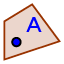# Isosceles Triangle Exploration

## DIRECTIONS:

1) Use the CIRCLE WITH CENTER THROUGH POINTtool to construct a circle with center A that passes through B. 2) Select the POINT ON OBJECTtool. With this tool selected, touch the circle in 2 different spots to plot two different points, C and D, on the circle itself.

3) Use the POLYGONtool to construct the triangle ACD. How would you classify this triangle by its sides? Why is this?

4) Select the MOVEtool. Now touch one blue segment that serves as a side of this triangle. In the style bar that appears, select the "Aa" icon. Check "Value" to show the length of this segment. Repeat this action for the other 2 sides as well. 5) Use the ANGLEtool to find and display the measures of all 3 angles of this triangle.

## 6)

What do you notice about two of the angles? (Be sure to select the MOVE tool again and move all 4 points around!)

## 7)

What can you say about all isosceles triangle that is not always true about other triangles? This is called the Isosceles Triangle Theorem.

Select all that apply
• A
• B
• C
• D